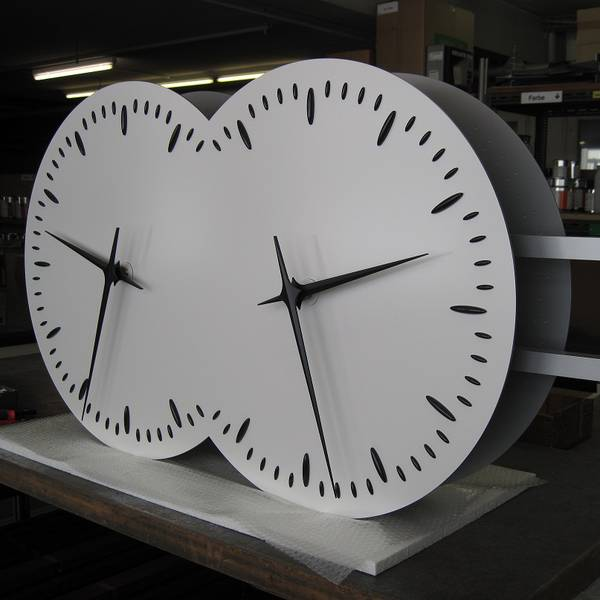# Tik-Tok Tik-TokTwo clocks $A$ and $B$ of radii $r$ and $R$ respectively, with $r, show the same time.

The tip of the minute hand of which clock will move with greater speed?

×